Guru

# Draw different pairs of circles. How many points does each pair have in common? What is the maximum number of common points? Q.1

• 0

sir what is the best way for solving the question of class 9th chapter of Circles chapter of question no.1 of math of exercise 10.3 of math, what is the best way for solving the question of class 9th ncert book of question no.1 Draw different pairs of circles. How many points does each pair have in common? What is the maximum number of common points?

Share

1.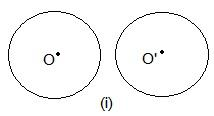In these two circles, no point is common.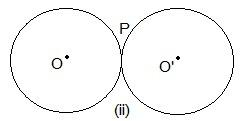Here, only one point “P” is common.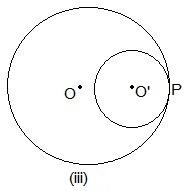Even here, P is the common point.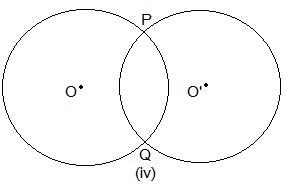Here, two points are common which are P and Q.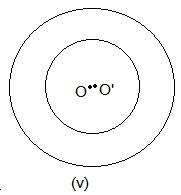No point is common in the above circle.

• 0